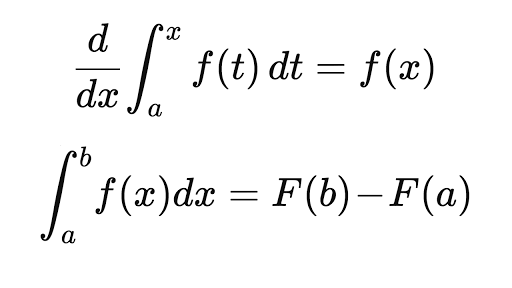# Antiderivatives & The Fundamental Theorem Of Calculus Assessment Test

10 Questions | Total Attempts: 116SettingsWe apply the derivative rules in reverse when we are finding antiderivatives, which are functions whose derivatives are ƒ. Take this assessment test to find out more.

Related Topics
• 1.
The word indefinite integral is synonymous to...
• A.

Solution

• B.

Limit

• C.

Antiderivative

• D.

Integration

• 2.
Diifferential equations in x and y is an equation that involves x,y and derivatives of y.
• A.

True

• B.

False

• C.

Depends on some factors

• D.

Not defined

• 3.
Use the properties of summation to evaluate:    24    Σ (5i - 4) =    i=1
• A.

614

• B.

589

• C.

615

• D.

616

• 4.
Use the properties of summation to evaluate:     10     Σ (2i + 3)     i=1
• A.

70

• B.

140

• C.

210

• D.

290

• 5.
Evaluate the sum.     7     Σ =     i=1
• A.

28

• B.

35

• C.

39

• D.

34

• 6.
Derivatives and integral, are essentially inverse operations on functions.
• A.

True

• B.

False

• C.

Maybe

• D.

Not defined

• 7.
Find all antiderivatives of f ( x ) = 1/(1 + x ) + 2 cos(2 x ).
• A.

F( x ) = log(1 + x ) + sin(2 x )

• B.

F( x ) = log(2 + x ) + sin(2 x )

• C.

F( x ) = log(1 + x ) + sin(1 x )

• D.

F( x ) = log(2 + x ) + sin(3 x )

• 8.
Which Greek letter is used for sigma notation?
• A.

ξ

• B.

Σ

• C.

φ

• D.

Φ

• 9.
The connection between differential calculus and integral calculus is stated in a theorem that is appropriately called the...
• A.

Mean Value theorem

• B.

Pythagorean theorem

• C.

Binomial theorem

• D.

Fundamental theorem of calculus

• 10.
There are _____ types of fundamental theorem of calculus.
• A.

2

• B.

3

• C.

4

• D.

5